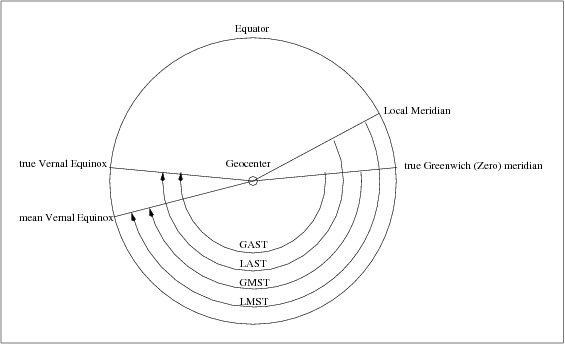If you wish to contribute or participate in the discussions about articles you are invited to join Navipedia as a registered user

# Sidereal Time

Jump to navigation Jump to searchFundamentals
Title Sidereal Time
Author(s) J. Sanz Subirana, J.M. Juan Zornoza and M. Hernández-Pajares, Technical University of Catalonia, Spain.
Level Basic
Year of Publication 2011

The reference is the Vernal Equinox, or the Aries point, which is defined as the intersection between the equator and the ecliptic plane [footnotes 1]. Two types of Aries point can be considered depending on which equator plane is considered (i.e, the mean equator or the true equator).

According to the previous definitions, four classes of sidereal times are introduced:

• Local "Apparent" Sidereal Time (LAST)($\Theta$): is the hour angle of the "true" Aries point (from local meridian) [footnotes 5].
• Greenwich "Apparent" Sidereal Time (GAST)($\Theta_{_G}$): is the hour angle of "true" Aries point, from Greenwich meridian.
• Local Mean Sidereal Time (LMST)($\theta$): the same that LAST with the mean equinox.
• Greenwich Mean Sidereal Time (GMST)($\theta_{_G}$): the same that GAST with the mean equinox.

The Figure 2 summaries these four sidereal times.Figure 2: Different sidereal Times (from [Seeber, 1993]) 

GAST and GMST are given by equations (3) and (4) in CEP to ITRF, respectively. Local and Greenwich Sidereal Times differ by the longitude $\displaystyle \lambda$ of the local meridian. The difference between Apparent and Mean Sidereal Times is called Equation of Equinoxes (where $\displaystyle \alpha_E$ is given by equation (7) in CEP to ITRF):

$\begin{array}{lcl} GMST\,-\,LMST\,=\,GAST\,-\,LAST\,=\,\lambda \\ GMST\,-\,GAST\,=\,LMST\,-\,LAST\,=\,\alpha_E \end{array} \qquad \mbox{(1)}$